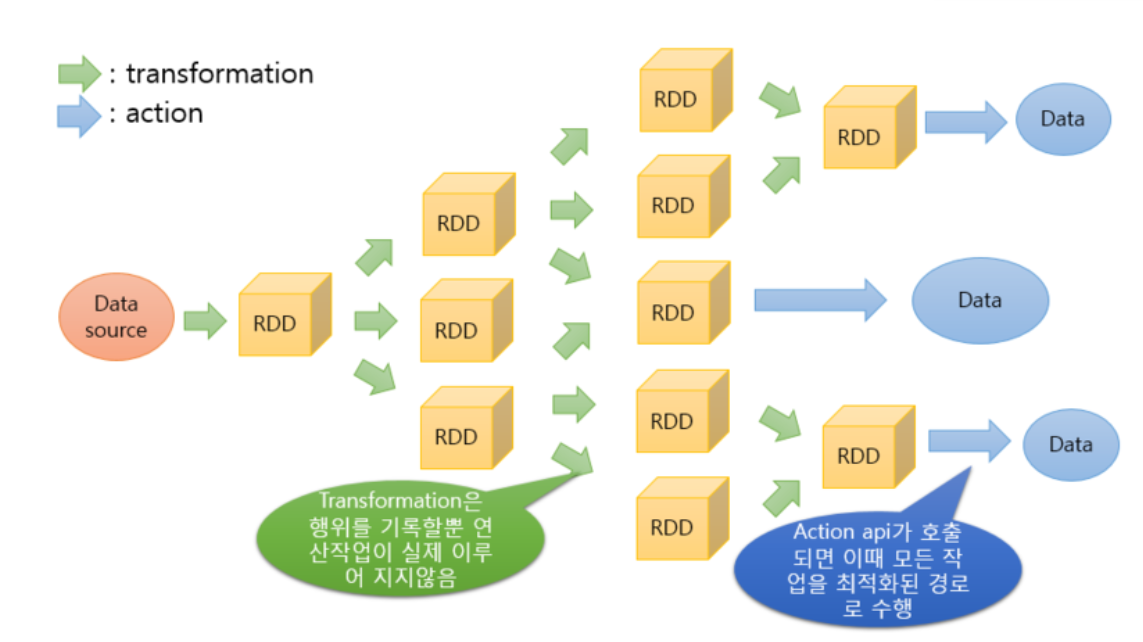# Spark Basic Operations

dougieduk·2022년 4월 10일
0

# Important Terms

### RDD : Resilient Distributed Dataset

• Basic unit of calculation for Spark (It's like an API for controling Spark)
• a read-only, fault-tolerant partitioned collection of records
• Lineage: User's commands are divided into multiple RDDs
• Directed Acrylic Graph : Actions are carried out one-way### Action : Spark operation that produces a local object

• Lazy Evaluation : Spark does not carry out calculations unless an Action is carried out

### Spark Job : Sequence of transformations on data with a final action

See how pyspark processes iterative/interactive processes

# Creating an RDD

• sc.parallelize(array) : create RDD of elements of array (or list)
• sc.textFile({path to file}): create RDD of lines from file

# RDD Transformation

• filter(lambda x: x%2 ==0) : Discard False elements
• map(labmda x: x*2) : multiply each RDD element by 2
• map(lambda x:x.split()) : split each string into words
• flatMap(lambda x: x.split()) : split each string into words and flatten sequence
• sample(withReplacement=True, 0.25) : create a sample of 25% of elements with replacement
• union(rdd) : append rdd to existing RDD
• distinct() : remove duplicates in RDD
• sortBy(lambda x:x, ascending=False) : sort elements in desceding order

# RDD Actions

• collect() : convert RDD to in-memory list
• take(3) : first 3 elements of RDD
• top(3) : top 3 elements of RDD (think about when you are carrying out SortBy actions)
• takeSample(withReplacement=True, 3) : create sample of 3 elements with replacement
• sum() : find element sum(assumes numeric elements)
• mean() : find element mean(assumes numeric elements)
• stdev() : find element deviation (assumes numeric elements)